# 程序员必备的基本算法：递归详解

### 前言

• 什么是递归？
• 递归的特点
• 递归与栈的关系
• 递归应用场景
• 递归解题思路
• leetcode案例分析
• 递归可能存在的问题以及解决方案

github地址，感谢每一颗Star

github.com/whx123/Java…

### 什么是递归？

``````public int sum(int n) {
if (n <= 1) {
return 1;
}
return sum(n - 1) + n;
}

### 递归的特点

• 自身调用：原问题可以分解为子问题，子问题和原问题的求解方法是一致的，即都是调用自身的同一个函数。
• 终止条件：递归必须有一个终止的条件，即不能无限循环地调用本身。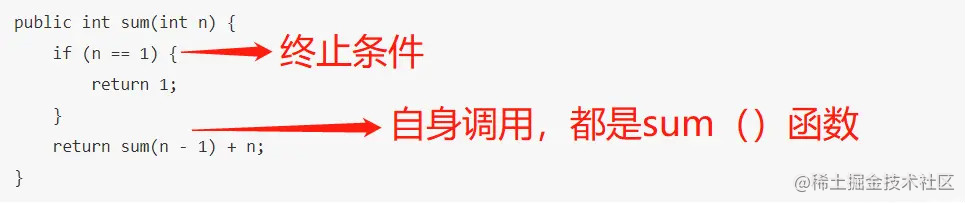### 递归与栈的关系

• 计算sum（5）时，先sum（5）入栈，然后原问题sum（5）拆分为子问题sum（4），再入栈，直到终止条件sum（n=1）=1，就开始出栈。
• sum（1）出栈后，sum（2）开始出栈，接着sum（3）。
• 最后呢,sum（1）就是后进先出，sum（5）是先进后出，因此递归过程可以理解为栈出入过程啦~

### 递归的经典应用场景

• 阶乘问题
• 二叉树深度
• 汉诺塔问题
• 斐波那契数列
• 快速排序、归并排序（分治算法也使用递归实现）
• 遍历文件，解析xml文件

### 递归解题思路

• 第一步，定义函数功能
• 第二步，寻找递归终止条件
• 第二步，递推函数的等价关系式

#### 1.定义函数功能

``````//n的阶乘（n为大于0的自然数）
int factorial (int n){

}

#### 2.寻找递归终止条件

``````//n的阶乘（n为大于0的自然数）
int factorial (int n){
if(n==1){
return 1;
}
}

#### 3.递推函数的等价关系式

``````int factorial (int n){
if(n==1){
return 1;
}
return n * factorial(n-1);
}

### leetcode案例分析

``````     4
/   \
2     7
/ \   / \
1   3 6   9

``````     4
/   \
7     2
/ \   / \
9   6 3   1

1. 定义函数功能

``````//翻转一颗二叉树
public TreeNode invertTree(TreeNode root) {
}

/**
* Definition for a binary tree node.
* public class TreeNode {
*     int val;
*     TreeNode left;
*     TreeNode right;
*     TreeNode(int x) { val = x; }
* }
*/

2.寻找递归终止条件

``````//翻转一颗二叉树
public TreeNode invertTree(TreeNode root) {
if(root==null || (root.left ==null && root.right ==null)){
return root;
}
}

3. 递推函数的等价关系式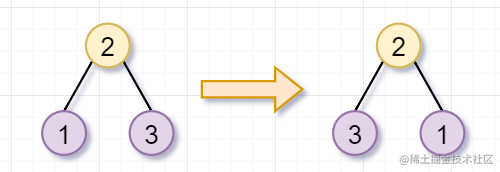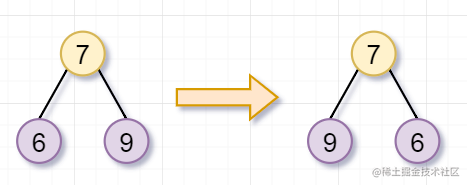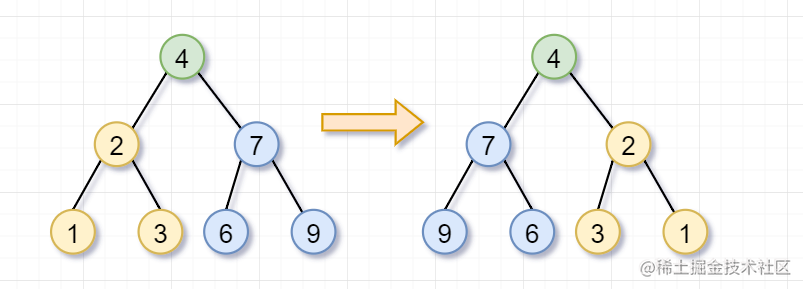``````invertTree（root）= invertTree（root.left） + invertTree（root.right）;

``````//翻转一颗二叉树
public TreeNode invertTree(TreeNode root) {
if(root==null || (root.left ==null && root.right ==null){
return root;
}
//翻转左子树
TreeNode left = invertTree(root.left);
//翻转右子树
TreeNode right= invertTree(root.right);
}

`````` root.left = right;
root.right = left;

``````class Solution {
public TreeNode invertTree(TreeNode root) {
if(root==null || (root.left ==null && root.right ==null)){
return root;
}
//翻转左子树
TreeNode left = invertTree(root.left);
//翻转右子树
TreeNode right= invertTree(root.right);
//左右子树交换位置~
root.left = right;
root.right = left;
return root;
}
}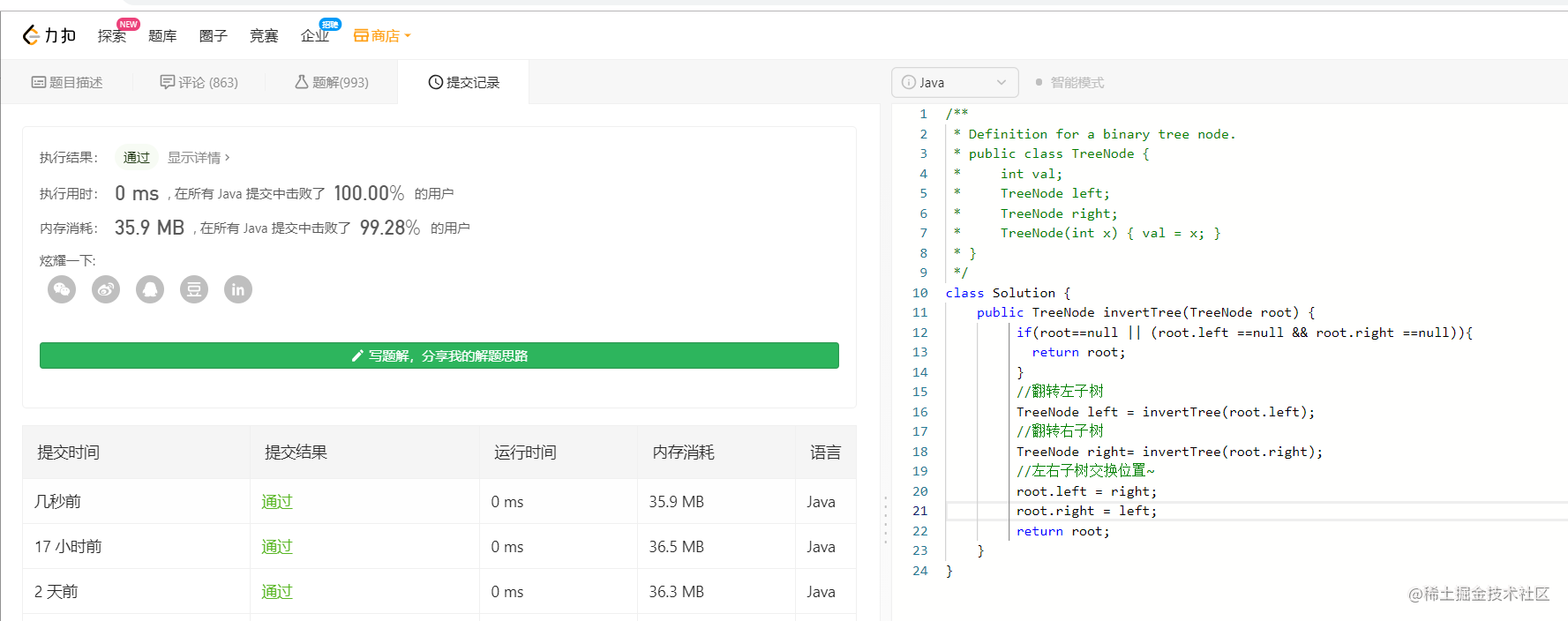### 递归存在的问题

• 递归调用层级太多，导致栈溢出问题
• 递归重复计算，导致效率低下

#### 栈溢出问题

• 每一次函数调用在内存栈中分配空间，而每个进程的栈容量是有限的。
• 当递归调用的层级太多时，就会超出栈的容量，从而导致调用栈溢出。
• 其实，我们在前面小节也讨论了，递归过程类似于出栈入栈，如果递归次数过多，栈的深度就需要越深，最后栈容量真的不够咯

``````/**
* 递归栈溢出测试
*/
public class RecursionTest {

public static void main(String[] args) {
sum(50000);
}
private static int sum(int n) {
if (n <= 1) {
return 1;
}
return sum(n - 1) + n;
}
}

``````Exception in thread "main" java.lang.StackOverflowError
at recursion.RecursionTest.sum(RecursionTest.java:13)

#### 重复计算，导致程序效率低下

``````class Solution {
public int numWays(int n) {
if (n == 0){
return 1;
}
if(n <= 2){
return n;
}
return numWays(n-1) + numWays(n-2);
}
}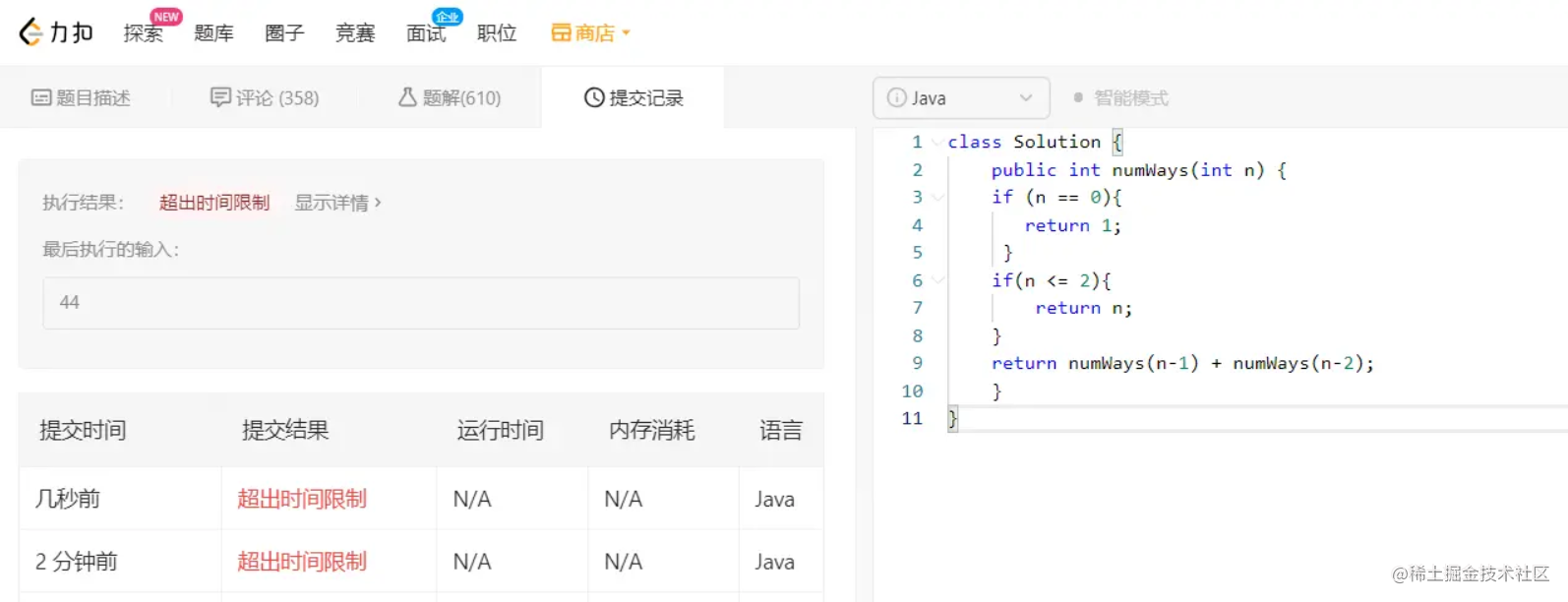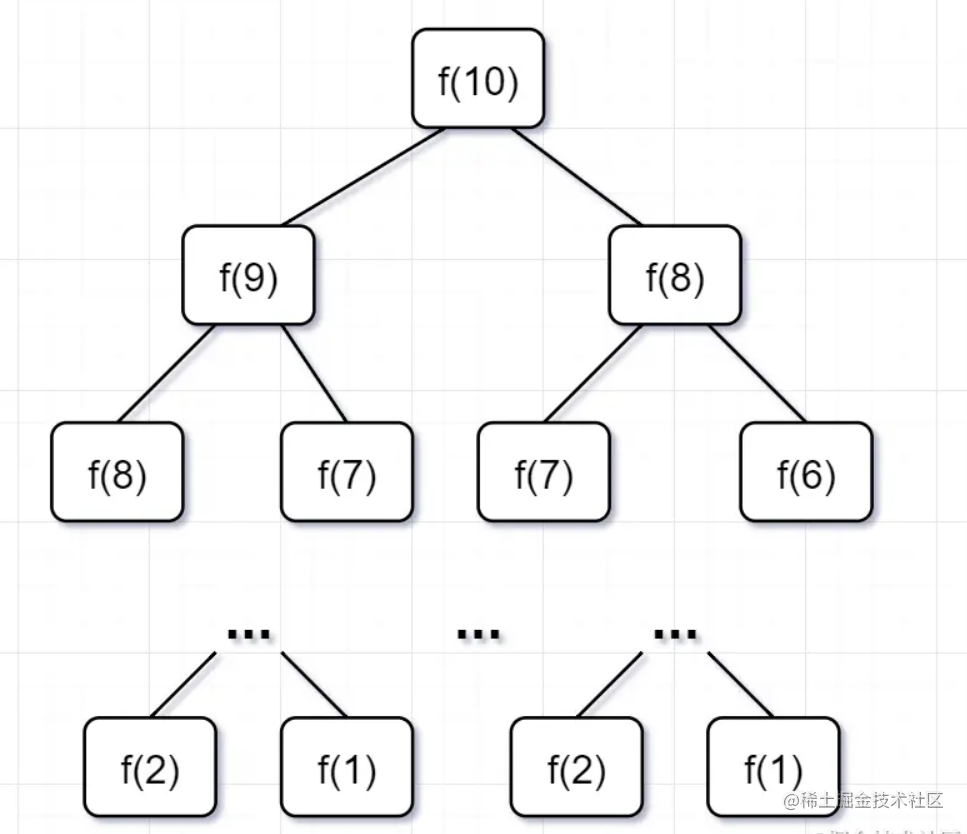• 要计算原问题 f(10)，就需要先计算出子问题 f(9) 和 f(8)
• 然后要计算 f(9)，又要先算出子问题 f(8) 和 f(7)，以此类推。
• 一直到 f(2) 和 f(1），递归树才终止。

• 一个子问题时间 = f（n-1）+f（n-2），也就是一个加法的操作，所以复杂度是 O(1)
• 问题个数 = 递归树节点的总数，递归树的总结点 = 2^n-1，所以是复杂度O(2^n)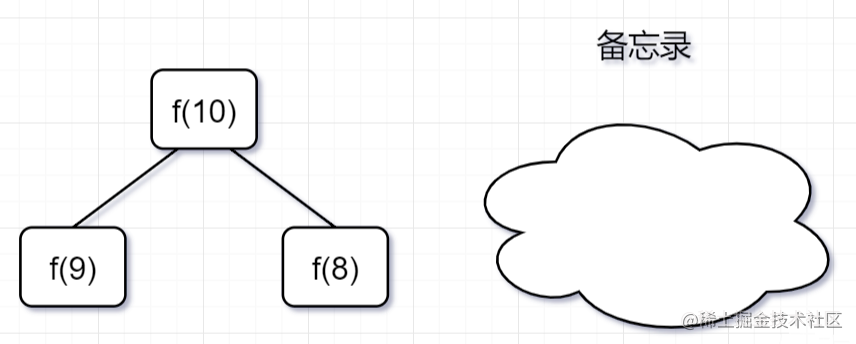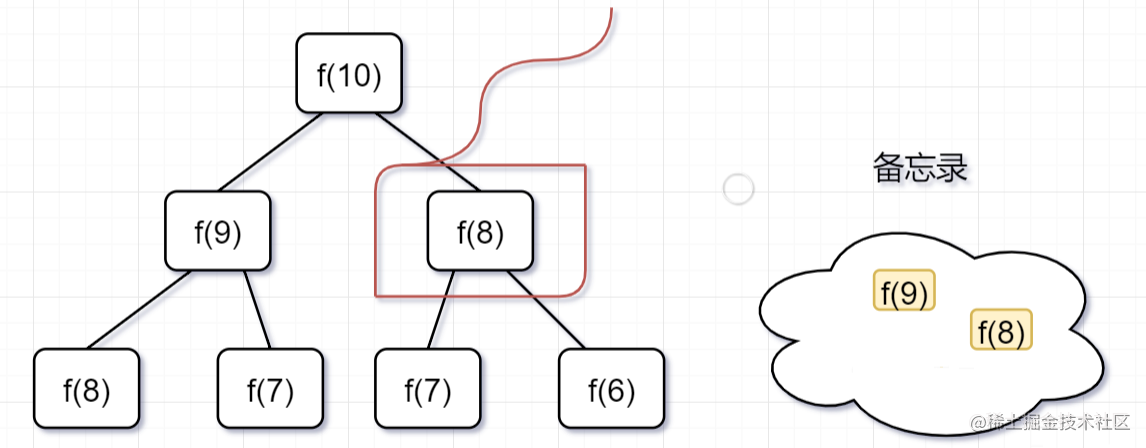``````public class Solution {
//使用哈希map，充当备忘录的作用
Map<Integer, Integer> tempMap = new HashMap();
public int numWays(int n) {
// n = 0 也算1种
if (n == 0) {
return 1;
}
if (n <= 2) {
return n;
}
//先判断有没计算过，即看看备忘录有没有
if (tempMap.containsKey(n)) {
//备忘录有，即计算过，直接返回
return tempMap.get(n);
} else {
// 备忘录没有，即没有计算过，执行递归计算,并且把结果保存到备忘录map中，对1000000007取余（这个是leetcode题目规定的）
tempMap.put(n, (numWays(n - 1) + numWays(n - 2)) % 1000000007);
return tempMap.get(n);
}
}
}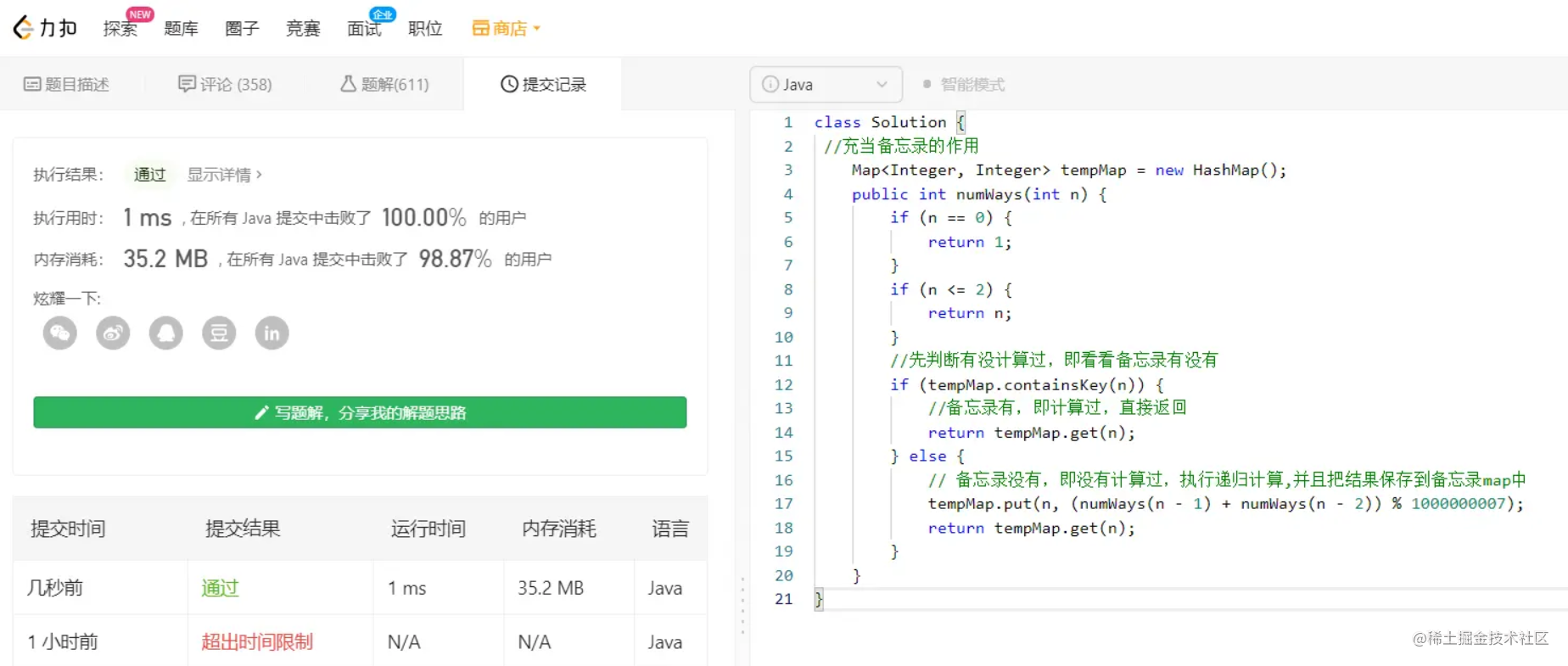### 更多干货

• 更多干货，关注公众号
• 回复pdf，获取学习电子书